# 生成对抗网络的简单介绍（TensorFlow 代码）

## 原文地址：

An introduction to Generative Adversarial Networks (with code in TensorFlow)

## 引言

“There are many interesting recent development in deep learning…The most important one, in my opinion, is adversarial training (also called GAN for Generative Adversarial Networks). This, and the variations that are now being proposed is the most interesting idea in the last 10 years in ML, in my opinion.” – Yann LeCun

## 判别模型与生成模型

• 判别模型学习将输入数据（x）映射到某个所需输出类标签（y）的函数。从概率出发，它们直接学习条件分布 Py|x $P（y | x）$
• 生成模型尝试同时学习输入数据和标签的联合概率，即 Pxy $P（x，y）$。这可以通过贝叶斯规则转换为 Py|x $P（y | x）$进行分类，但生成能力也可以用于别的东西，例如创建可能的新 xy $（x，y）$ 样本。

## 生成对抗网络

GANs是一个有趣的想法，由德国蒙特利尔大学的Ian Goodfellow（现OpenAI）领导的一组研究人员于2014年首次推出。 GAN的主要思想是拥有两个竞争的神经网络模型。 一个将噪声数据作为输入，并产生样本（所谓的生成器）。 另一个模型（称为判别器）从生成器和训练数据接收样本，并且必须能够区分两个来源。 这两个网络进行连续的博弈，生成器学习产生越来越多的现实样本，鉴别器正在学习越来越好地区分生成的数据和实际数据。 这两个网络同时进行训练，最后的希望是竞争能够使生成器生成的样本与实际数据不可区分。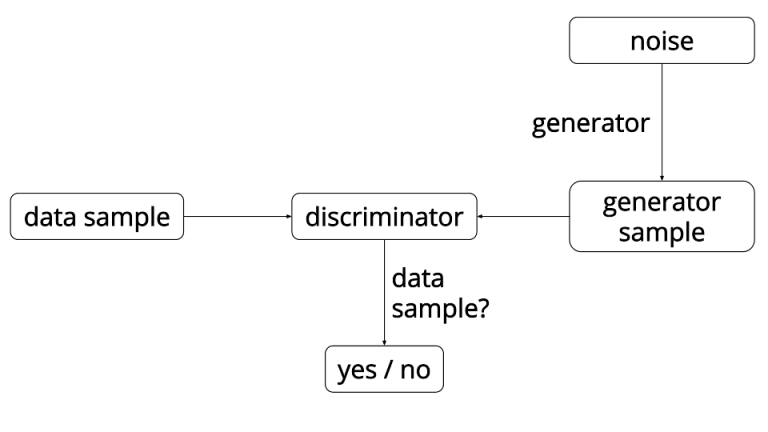GAN overview. Source: https://ishmaelbelghazi.github.io/ALIGenerated bedrooms. Source: “Unsupervised Representation Learning with Deep Convolutional Generative Adversarial Networks” https://arxiv.org/abs/1511.06434v2*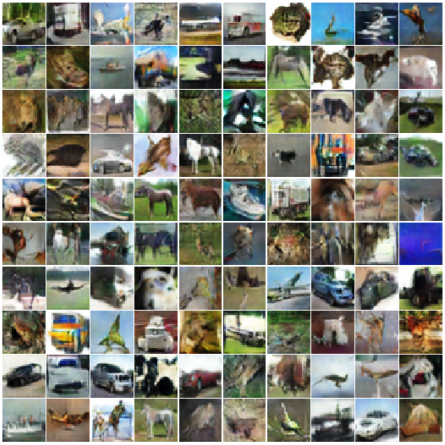Generated CIFAR-10 samples. Source: “Improved Techniques for Training GANs” https://arxiv.org/abs/1606.03498*

## 近似一维高斯分布

    class DataDistribution(object):
def init(self):
self.mu = 4
self.sigma = 0.5
def sample(self, N):
samples = np.random.normal(self.mu, self.sigma, N)
samples.sort()
return samples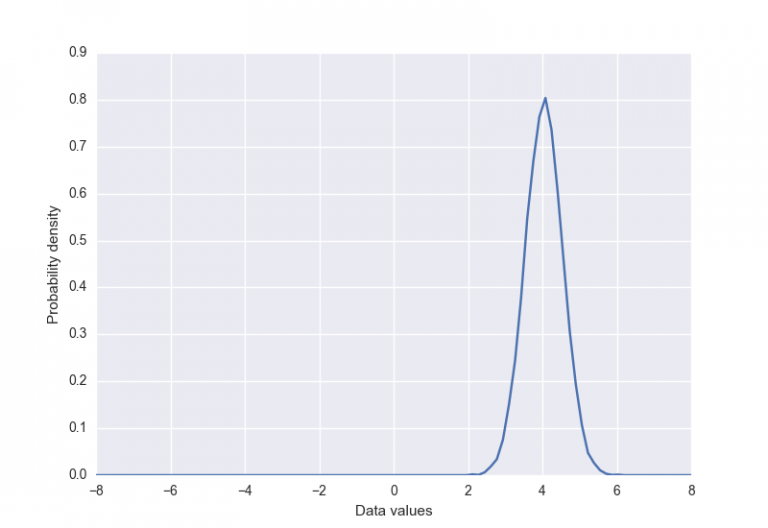class GeneratorDistribution(object):
def __init__(self, range):
self.range = range

def sample(self, N):
return np.linspace(-self.range, self.range, N) + \
np.random.random(N) * 0.01

    def generator(input, hidden_size):
h0 = tf.nn.softplus(linear(input, hidden_size, 'g0'))
h1 = linear(h0, 1, 'g1')
return h1

   def discriminator(input, hidden_size):
h0 = tf.tanh(linear(input, hidden_size * 2, 'd0'))
h1 = tf.tanh(linear(h0, hidden_size * 2, 'd1'))
h2 = tf.tanh(linear(h1, hidden_size * 2, 'd2'))
h3 = tf.sigmoid(linear(h2, 1, 'd3'))
return h3

  with tf.variable_scope('G'):
z = tf.placeholder(tf.float32, shape=(None, 1))
G = generator(z, hidden_size)

with tf.variable_scope('D') as scope:
x = tf.placeholder(tf.float32, shape=(None, 1))
D1 = discriminator(x, hidden_size)
scope.reuse_variables()
D2 = discriminator(G, hidden_size)

loss_d = tf.reduce_mean(-tf.log(D1) - tf.log(1 - D2))
loss_g = tf.reduce_mean(-tf.log(D2))

    def optimizer(loss, var_list):
initial_learning_rate = 0.005
decay = 0.95
num_decay_steps = 150
batch = tf.Variable(0)
learning_rate = tf.train.exponential_decay(
initial_learning_rate,
batch,
num_decay_steps,
decay,
staircase=True
)
loss,
global_step=batch,
var_list=var_list
)
return optimizer

vars = tf.trainable_variables()
d_params = [v for v in vars if v.name.startswith('D/')]
g_params = [v for v in vars if v.name.startswith('G/')]

opt_d = optimizer(loss_d, d_params)
opt_g = optimizer(loss_g, g_params)

  with tf.Session() as session:
tf.initialize_all_variables().run()

for step in xrange(num_steps):
# update discriminator
x = data.sample(batch_size)
z = gen.sample(batch_size)
session.run([loss_d, opt_d], {
x: np.reshape(x, (batch_size, 1)),
z: np.reshape(z, (batch_size, 1))
})

# update generator
z = gen.sample(batch_size)
session.run([loss_g, opt_g], {
z: np.reshape(z, (batch_size, 1))
})

https://youtu.be/mObnwR-u8pc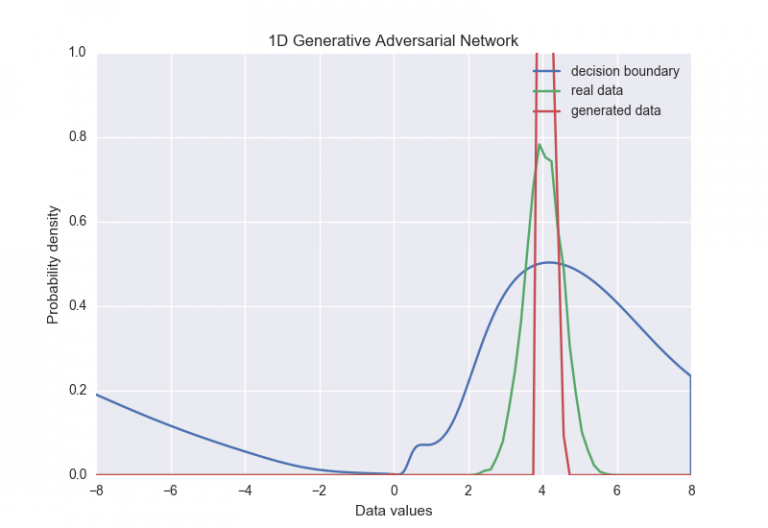## 提高样本多样性

• 取出判别器的一些中间层的输出。
• 将其乘以3D张量以产生矩阵（在下面的代码中大小为num_kernels x kernel_dim）。
• 在批量中的所有样本之间计算该矩阵中的行之间的L1距离，然后应用负指数。
• 样本的minibatch 特征是这些取幂距离的总和。
• 将原始输入连接到新创建的最小匹配特征的最小匹配层（前一个判别层的输出），并将其作为输入传递给判别的下一层。

  def minibatch(input, num_kernels=5, kernel_dim=3):
x = linear(input, num_kernels * kernel_dim)
activation = tf.reshape(x, (-1, num_kernels, kernel_dim))
diffs = tf.expand_dims(activation, 3) - \
tf.expand_dims(tf.transpose(activation, [1, 2, 0]), 0)
abs_diffs = tf.reduce_sum(tf.abs(diffs), 2)
minibatch_features = tf.reduce_sum(tf.exp(-abs_diffs), 2)
return tf.concat(1, [input, minibatch_features])


https://youtu.be/0r3g7-4bMYU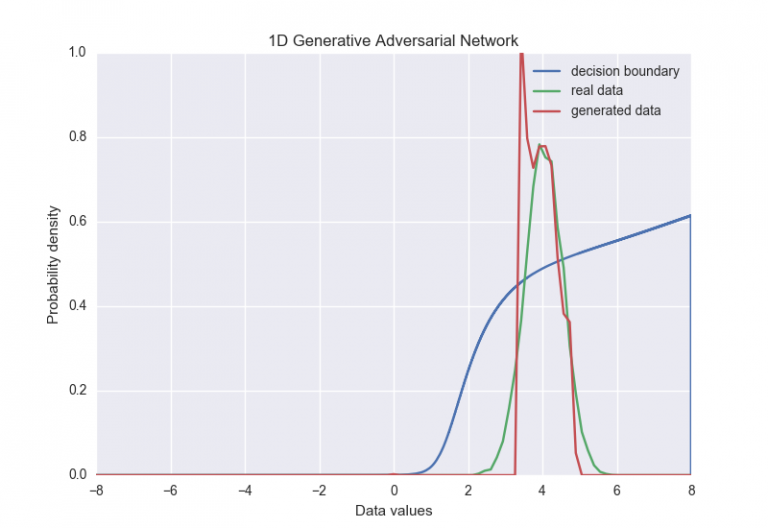minibatch discrimination的最后一点是，使批量大小作为超参数更为重要。 在我们的示例中，我们不得不保持批量相当小（不到16个左右）进行训练。 也许仅仅限制对每个距离测量有贡献的样本数量，而不是使用完整批次，但是再次调整另一个参数就足够了。

## 更多信息

• Unsupervised Representation Learning with Deep Convolutional Generative Adversarial Networks
• InfoGAN: Interpretable Representation Learning by Information Maximizing Generative Adversarial Nets
• Improved Techniques for Training GANs

## 原文地址：

An introduction to Generative Adversarial Networks (with code in TensorFlow)

10-161万+08-061119
02-14
06-306762
12-261万+
05-2611万+
07-041930
09-271万+
03-31
08-232153
07-312万+
05-24
01-081万+
09-26308
05-311021
03-021795